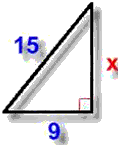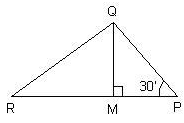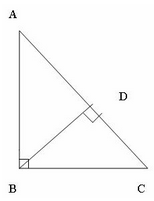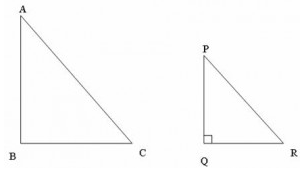Courses

# Test: Pythagoras Theorem

## 15 Questions MCQ Test Mathematics (Maths) Class 10 | Test: Pythagoras Theorem

Description
This mock test of Test: Pythagoras Theorem for Class 10 helps you for every Class 10 entrance exam. This contains 15 Multiple Choice Questions for Class 10 Test: Pythagoras Theorem (mcq) to study with solutions a complete question bank. The solved questions answers in this Test: Pythagoras Theorem quiz give you a good mix of easy questions and tough questions. Class 10 students definitely take this Test: Pythagoras Theorem exercise for a better result in the exam. You can find other Test: Pythagoras Theorem extra questions, long questions & short questions for Class 10 on EduRev as well by searching above.
QUESTION: 1

### Which of the following cannot be the sides a right triangle?​

Solution:

H= P+ B2
H2 = 81
P+ B= 25 + 49 = 74
H ≠ P2+B2.Therefore the sides cannot form a right triangle.

QUESTION: 2

### In a triangle ABC, AC= √180, AB = 6, BC = 12. What is ∠B = ?

Solution:

We have AB2=36 and BC2=144
Adding both the squares we get
AB2+BC2=36+144=180
=AC2
So , AC is the hypotenuse according to pythagoras theorem , So the angle opposite to it which is B is 90 degrees.

QUESTION: 3

### From the given figure, find the unknown x.​

Solution:

Since the triangle is a right angled triangle,
Applying Pythagoras Theorem,
H2=P2+B2
152=P2+92
P2=225-81=144
P=12

QUESTION: 4

Three squares are based on the sides of a right angled triangle. The area of the two smaller ones are 144 sq. cm and 256 sq. cm. What is the area of the third one?​

Solution:
QUESTION: 5

What is the diagonal length of a TV screen whose dimensions are 80 x 60 cm?

Solution:

We have a TV which is a rectangle whose adjacent sides form an angle of 90 degrees so The triangle formed by the two sides and a diagonal will be a right angle. So, Applying Pythagoras Theorem,
(Diagonal)2=802+602=6400+3600=10000
Diagonal=100cm

QUESTION: 6

Which of the following is a Pythagorean triplet ?​

Solution:

A Pythagorean triple consists of three positive integers a, b, and c, such that a² + b² = c².

a) 432 = 1849

362+182 = 1620

b) 252  = 625

15+ 202 =625

c) 132 = 169

32 + 122 = 153

d) 262 = 676

242 + 252 = 1201

so option b is correct

QUESTION: 7

QM ⊥ RP and PR2 – PQ2 = OR2. If∠QPM = 30°, Then ∠MQR isSolution:

In triangle PMQ
Angle M = 90°
Angle P = 30°
Angle QMP + Angle MPQ + Angle PQM = 180 degree ( By angle sum property )
90 + 30 + Angle PQM = 180
Angle PQM = 180 - 120
Angle PWM = 60 Degree
QM divides Angle PQR into two equal parts .
Therefore Angle MQR = 30°

QUESTION: 8

In the above figure, AB = c, BC = a, AC = b, AD = y, DB = p. Check which of the following options is correct ?​Solution:
QUESTION: 9

In right triangled ABC right angled at B, a line DE is drawn through the mid point D of AB and parallel to BC. If AB = 9 cm, BC = 12 cm. AE = ?​

Solution:

In a triangle ABC in which angle B = 90 degree
So, AB is height of the given triangle = 9 cm
Base of triangle i.e, BC = 12 cm
DE is || to BC
D is the mid point of side AB and E is the mid point of side AC
By applying pythagoras
AC2 = AB2 + BC2
AC2 = 9^2 + 12^2
AC2 = 81 + 144
AC2 = 225
AC = root under 225
AC = 15 cm
E is the mid point of AC
Therefore AE = AC / 2
AE = 15 / 2
AE = 7.5 cm

QUESTION: 10

A boy is trying to catch fish sitting at a height of 12 m from the surface of the water. A big fish is at a horizontal distance of 5 m from him. What should be the length of his string to get the fish?

Solution:
QUESTION: 11

Three numbers form a Pythagorean triplet. Two of them are 15 and 17 where 17 is the largest of them. The third number is

Solution:
QUESTION: 12

Triangle PQR is an isosceles right triangle right angled at Q. If PR = √50. What is the value of each of the equal sides?​

Solution:
QUESTION: 13

The length of an altitude of an equilateral triangle of side a is:

Solution:
QUESTION: 14

Two friends A and B start from the same point in the Eastern and Northern directions at the same time. How far are they from each other when A has travelled 5 km and B has travelled 12 km. distance?​

Solution:
QUESTION: 15

Two similar right triangles ABC and PQR are as shown in the figure.If AB = √3 , PQ = √3/2,BC = 1. Find PR = ?Solution: